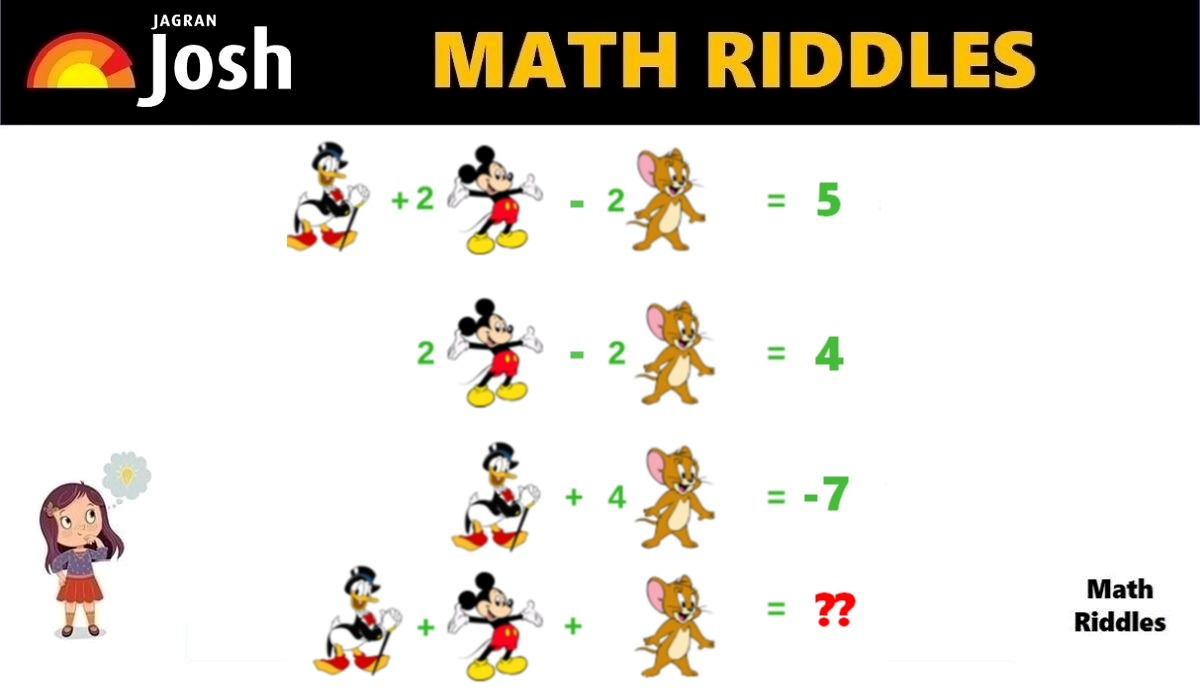# Math Riddles With Answers: Solve This Donald Duck, Jerry, Mickey Mouse Math Puzzle in 20 Seconds

In this Math Puzzle, we have to find is the values of Donald Duck, Jerry, and Mickey Mouse to solve this hard math riddle. Are you a math genius? Only 1% with a high IQ can solve this tricky math puzzle in 20 seconds.Math Riddles With Answers: Solve This Donal Duck, Jerry, Mickey Mouse Math Puzzle in 20 Seconds

In this Math Puzzle, we have to find the values of Donald Duck, Jerry, and Mickey Mouse to solve this hard math riddle. Are you a math genius? Only 1% with a high IQ can solve this tricky math puzzle in 20 seconds.

Share this math puzzle with your friends, family, and students. These math riddles are a great source for solving math problems in a fun way. These math puzzles are for all grades and ages. Try to test your mathematical knowledge and visual perception with this math puzzle. Let us solve this math picture puzzle within 20 seconds.

If you love solving cryptic math puzzles, give this math puzzle a try! You have 20 seconds to find the correct answer.

## Math Riddles with Answers: Solution

Explanation

Let us first summarize the equations here:

1 Donald Duck + 2 Mickey Mouse – 2 Jerry = 5 …………… (Row 1)

2 Mickey Mouse – 2 Jerry = 4 ……………. (Row 2)

1 Donald Duck + 4 Jerry = -7 …………… (Row 3)

1 Donald Duck + 1 Mickey Mouse + 1 Jerry = ?? ………. (Row 4)

So, we need to find the values of Donald Duck, Jerry, and Mickey Mouse to solve Row 4.

Can You Solve This Tricky Flower Math Riddle In 20 Seconds?

 Benefits of Math Puzzles and Riddles: Math puzzles make mathematics fun. Math puzzles help children grasp mathematical concepts easily. Math puzzles help in enhancing calculations and improving solving skills. Math puzzles help in developing logical reasoning.

Let us begin solving!

In Row 1 equation, we have 1 Donald Duck + 2 Mickey Mouse – 2 Jerry = 5

Now, if we notice the Row 2 equation, we have 2 Mickey Mouse – 2 Jerry = 4

So, we can substitute the information from Row 2 in the Row 1 equation.

We now get,

=> 1 Donald Duck + 2 Mickey Mouse – 2 Jerry = 5

=> 1 Donald Duck + 4 = 5

=> 1 Donald Duck = 5 – 4

Hence, the value of 1 Donald Duck = 1

Math Riddles With Answers: Can You Find The Value Of Lemon In 20 Seconds?

Moving on,

We shall use the value of 1 Donald Duck in the Row 3 equation to solve further.

In Row 3, we have 1 Donald Duck + 4 Jerry = -7

=> 1 + 4 Jerry = -7

=> 4 Jerry = -7 -1

=> 4 Jerry = -8

=> 1 Jerry = -8 / 4

=> 1 Jerry = -2

Hence, the value of 1 Jerry = -2

Test Your Observation Skills! Find These Hidden Objects In This Picture Puzzle In 11 Seconds?

Moving on, we shall use the value of 1 Jerry in Row 2 to solve further.

In Row 2, we have 2 Mickey Mouse – 2 Jerry = 4

=> 2 Mickey Mouse – (-2) = 4

=> 2 Mickey Mouse + 4 = 4

=> 1 Mickey Mouse = 4 – 4

=> 1 Mickey Mouse = 0

Hence, the value of 1 Mickey Mouse = 0

Genius Alert! Can You Find The Hidden Fish In This Picture Puzzle In 11 Seconds?

Now, we shall solve Row 4 as we have found the values of Donald Duck, Jerry, and Mickey Mouse.

In Row 4, we have 1 Donald Duck + 1 Mickey Mouse + 1 Jerry = ??

=> 1 + 0 + (-2) = ??

=> 1 + 0 – 2 = ??

=> -1

Hence, the final answer for this math puzzle is -1

Great Work!

## Tell us in comments: Did you solve this math puzzle correctly in 20 seconds?

Check out more amazing tricky math puzzles!

Math Riddles With Answers: Can You Find The Value of Each Candy in 20 Seconds?

Math Riddles With Answers: Can You Solve This Tricky Math Rebus Puzzle 20 Seconds?

Math Riddles With Answers: Can You Find The Value of Burger, Fries, Coke in 20 Seconds?

Math Riddles With Answers: Can You Find The Value of Each Fruit in 20 Seconds?

Get the latest General Knowledge and Current Affairs from all over India and world for all competitive exams.
खेलें हर किस्म के रोमांच से भरपूर गेम्स सिर्फ़ जागरण प्ले पर